## Social Question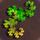# A group of people all randomly pick another member in the group. How many people are not picked by anybody? {see details}?

Asked by PhiNotPi (12677) July 7th, 2012

Anybody else like my use of curly brackets in the title? I’m trying to be different.

There is a group of 100 people at a party, numbered 1–100. Each person independently picks a random integer in the same range, excluding their own number. If a person has his assigned number picked by at least one person, then he gets cake. On average, how many people do not have their number picked by anybody?

Because of the well known birthday paradox, it likely that at least two people out of the group will pick the same number, so it is very likely that at least one person will walk away with no cake.

A general solution to this problem would be some formula such that given X people at the party, it gives Y average number of people without cake.

I am interested in this problem because of the 80–20 rule. There is guaranteed to be a number N such that N% of the people received (100-N)% of the votes, and I wonder if that number will be 80 or 20.

This problem at first reminds me of the birthday paradox, but it is different in that the number of numbers that each person could potentially pick is not a constant 365, but varies with the size of the group.

I hope that I have explained this problem well enough. Feel free to ask any questions.

Observing members: 0Composing members: 0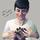The birthday problem is easier to solve than this because it’s not asking for the expected number of matching birthdays, it just asks whether there are matching birthdays. To do the same for your problem is also easy. The only way everybody’s number could be picked is if nobody picked any matching numbers. The probability of this is found as follows:

In the numerator we want the situation in which all the numbers are different, so 0 people miss out on cake. The first person’s number could be any of 100 numbers, the second person’s number could be any of 99… and this continues down the the last person, who has but one choice in order to avoid picking any numbers that anyone else has picked. So our numerator is 100! (factorial). Or in the general case, X!

The denominator is the total number of possible picks. In this case each person could pick any of the 100 numbers. So our denominator is 100^100. Or in the general case, X^X

This produces an incredibly tiny probability, on the order of 10^-43! So almost definitely some people will not get cake.

But how to find the expected value of people who don’t get cake…I can think of a lengthy, inefficient way to do it, and that’s by a weighted average. I’m not sure if there’s a better way.

We repeat the calculations above but with parameters changed so that our result will tell us the probability that exactly 1 person will not get cake. So we need 99 people all picking different numbers, and 1 person picking a number that’s the same as somebody else’s number.

Just like before, the first person has 100 choices, the second has 99… all down the the second to last person, who has 2 choices. But now the last person, instead of having 1 choice, has 99 choices. So our numerator is 100!/1! * (100–1). Our denominator is the same as before, and now we have the probability that exactly 1 person doesn’t get cake.

Now unfortunately we must repeat this for every number through 100. If Z is the number of people who don’t get cake, the formula for the numerator in each case is 100!/Z! * (100 – Z).

One we have each individual probability, we compute a weighted average by multiplying probability(Z) * Z and adding the results for Z = 1 through Z = 100. And this will find us the expected value we’re looking for.

Put in general terms, the formula you’re looking for is:

Y = Summation Z=1 though Z=X of (X!/Z!) * (X – Z) / X^X

If there’s an easier way to do this, I hope someone here will find it! My method wouldn’t be hard to do with a computer program for X of reasonable sizes.

Mariah (25876)“Great Answer” (2) Flag as…I want to correct what I wrote earlier… the summation should be from Z=0 through Z=X-1. Because it’s possible for 0 people to miss out on cake, and it’s possible for 99 people to miss out on cake, but it’s not possible for all 100 people to miss out, because at least one person’s number is going to get picked.

I’m also doubting my formula 100!/Z! * (100 – Z) because this formula does not reduce to the formula I found when Z = 0, which is just 100!. Instead it reduces to 100! * 100. I’m trying to find my error now.

Mariah (25876)“Great Answer” (1) Flag as…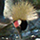Other distributions can be used to approximate Binomials (such as this) when N is large.

Copied from wikipedia
Poisson approximation

The binomial distribution converges towards the Poisson distribution as the number of trials goes to infinity while the product np remains fixed. Therefore the Poisson distribution with parameter λ = np can be used as an approximation to B(n, p) of the binomial distribution if n is sufficiently large and p is sufficiently small. According to two rules of thumb, this approximation is good if n ≥ 20 and p ≤ 0.05, or if n ≥ 100 and np ≤ 10.
Limiting distributions

Poisson limit theorem: As n approaches ∞ and p approaches 0 while np remains fixed at λ > 0 or at least np approaches λ > 0, then the Binomial(n, p) distribution approaches the Poisson distribution with expected value λ.[citation needed]

de Moivre–Laplace theorem: As n approaches ∞ while p remains fixed, the distribution of

{X-np \over \sqrt{np(1-p)\ }}

approaches the normal distribution with expected value 0 and variance 1.[citation needed] This result is sometimes loosely stated by saying that the distribution of X is asymptotically normal with expected value np and variance np(1 − p). This result is a specific case of the central limit theorem.

6rant6 (13692)“Great Answer” (2) Flag as…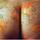What do you mean a person “picks” a random integer? Is the integer randomly selected or does the person do the picking?

wundayatta (58663)“Great Answer” (1) Flag as…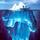Pardon me for revisiting a week-old thread. @PhiNotPi – The way I understand the game an integer in the range 1 to N is either (a) randomly assigned to; or (b) chosen by; each of N=100 people at a party. If you match at least one other person, you get cake. If you believe others are choosing randomly then case (b) reduces to case (a) unless players may communicate, in which case they should all agree on the same number in advance, guaranteeing cake for all.

Otherwise the probability of each number is 0.01 or 1% or 1/100. @Mariah does a variant of the “birthday calculation” based on 100 instead of 365, calculating the probability of failure p to yield the probability of success 1-p.

p = (99/100)*(98/100) *… *(1/100) = (N-1)! / N^(N-1) gives the probability that nobody else got your number, hence no cake for you. Fortunately p is so small your chances of getting cake are almost certain because 1-p is so nearly 1, i.e., certainty.

My calculator only goes to 92! (93! & higher “Not a number”) but close enough to make it easy to calculate 99!, which turns out to be 9.33×10^155
thus p (no cake) = 99! / 100^99
p = 99! / 10^198
p = 9.33×10(155 -198) = 9.33×10^-57

Since the same calculation applies to each player, pretty much everybody ends up almost always eating cake – a Hollywood ending! Even if you played constantly at once per second, you would expect to lose less than once per lifetime of the universe. Those are pretty good odds.

After many party-goers have bailed there are only M people left (including yourself) M<=N
p (no cake for you) = (99/100)*(98/100)… *( 100-M / 100 )
p = ( N-1)! / (100-M)! ) / N^(M-1)

With 50 people (N=100, M=50)
p = (99! / 50! ) / 100^49
Rewrite denominator 100^49 = 10^98
p = (9.33 / 3.04) x10^(155–64-98)
p = 3.07×10^-7
The chance of getting cake 1-p with only 50 people is still very close to 100% but you might lose once every few million games.

After the party dwindles to 15 people (N=100, M=15) it works out to
p = (99*98…86)/10^28 = .33 = 33%, giving you a ⅔ chance of getting cake.

With only 10 people
p = (99*98…91) / (100^9)
= (6.3×10^17) / 10^18 = .628, i.e., 63%
so p(cake) = 37%, which averages getting cake every 2.7 games. The situation has swung in the opposite direction.

For M=12 people it works out to p = 0.5031, just over a 50% chance you’ll miss out on cake.

gasman (11315)“Great Answer” (1) Flag as…Correction: Reviewing previous post I see what I calculated was probability that none of the numbers match, i.e., no winners. For a single individual’s chances, the factorials are replaced by powers of 99.

gasman (11315)“Great Answer” (1) Flag as…